Question

# Question 11 Ip Which statement is true of the internal energy (AU) of a system and...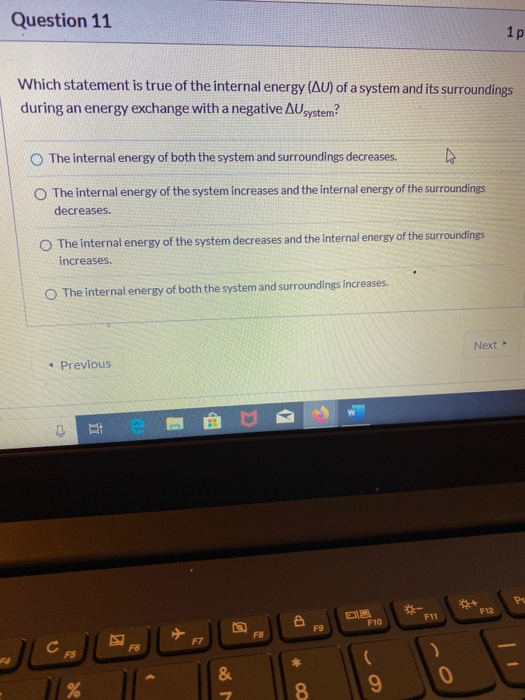Question 11 Ip Which statement is true of the internal energy (AU) of a system and its surroundings during an energy exchange with a negative Ausystem? The internal energy of both the system and surroundings decreases The internal energy of the system increases and the internal energy of the surroundings decreases. The internal energy of the system decreases and the internal energy of the surroundings increases. The internal energy of both the system and surroundings increases. Next → Previous CES co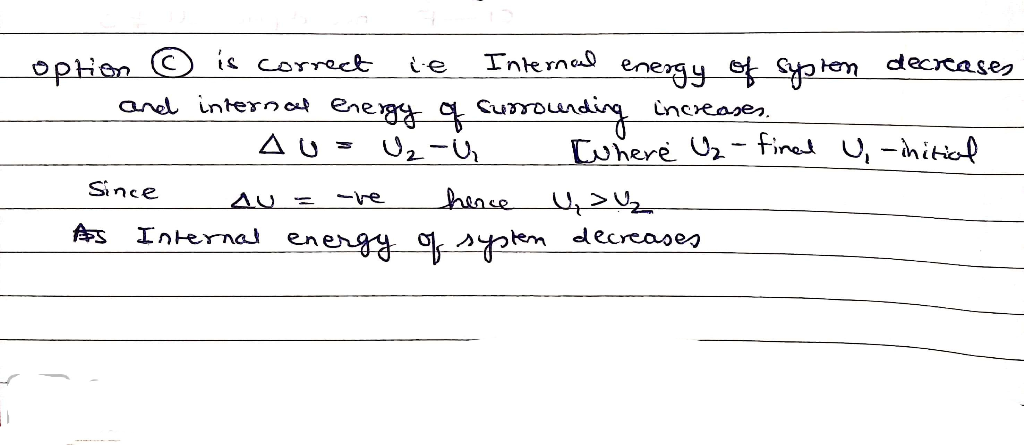Here is the solution of your question. If you have any doubt or need any clarification please comment in comment box and will definitely resolve your query. If you find useful please upvote it. Thanks in advance.

#### Earn Coins

Coins can be redeemed for fabulous gifts.

Similar Homework Help Questions
• ### Energy Units

Which of the following is true of the internal energy of a system and its surroundings during an energy exchange with a negative ?

• ### Which of the following statements is correct? The internal energy of a system increases when more...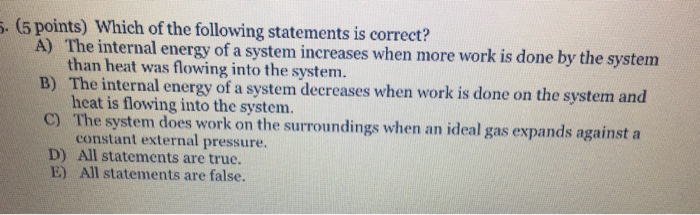Which of the following statements is correct? The internal energy of a system increases when more work is done by the system than heat was flowing into the system. The internal energy of a system decreases when work is done on the system and heat is flowing into the system. The system does work on the surroundings when an ideal gas expands against a constant external pressure. All statements are true. All statements are false.

• ### Describe a process in which the internal energy of some system increases as a result of...

Describe a process in which the internal energy of some system increases as a result of work done on the system by its surroundings. Be sure to identify all objects included in your system.

• ### 1. A gas absorbs 0.0 J of heat and then performs 30.8 J of work. The change in internal energy of the gas is a. 61....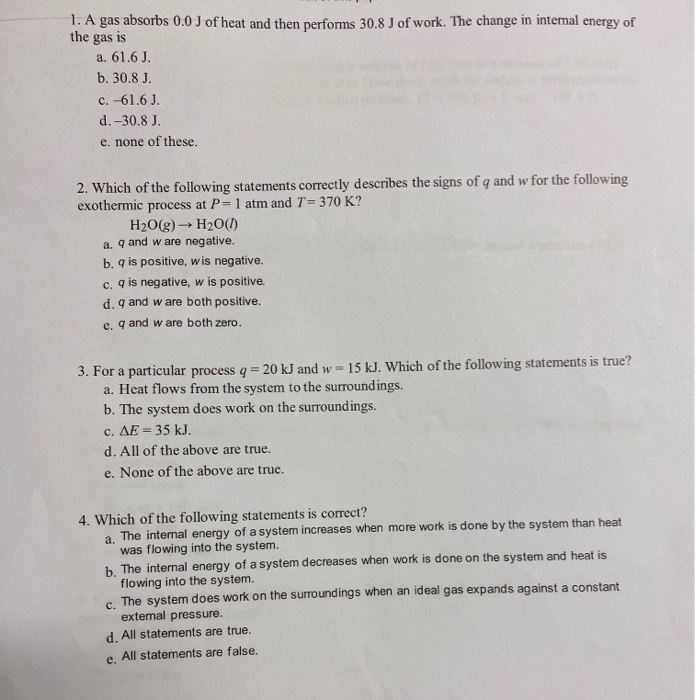1. A gas absorbs 0.0 J of heat and then performs 30.8 J of work. The change in internal energy of the gas is a. 61.6 J. b. 30.8 J. c. -61.6 J. d.-30.8 J. e. none of these 2. Which of the following statements correctly describes the signs of q and w for the following exothermic process at P= 1 atm and T = 370 K? H2O(g) → H2O(1) a. q and w are negative. b. is positive, wis...

• ### 2. A gaseous system absorbs 43 J of heat and increases its internal energy by 64...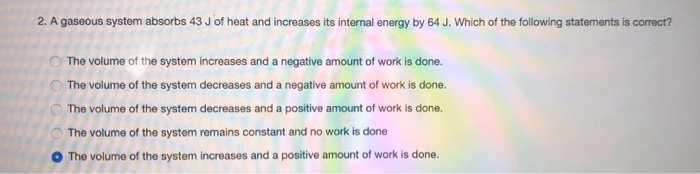2. A gaseous system absorbs 43 J of heat and increases its internal energy by 64 J. Which of the following statements is correct? The volume of the system increases and a negative amount of work is done. The volume of the system decreases and a negative amount of work is done. The volume of the system decreases and a positive amount of work is done. The volume of the system remains constant and no work is done The volume...

• ### What is the sign of AU as the system of ideal gas goes from point A...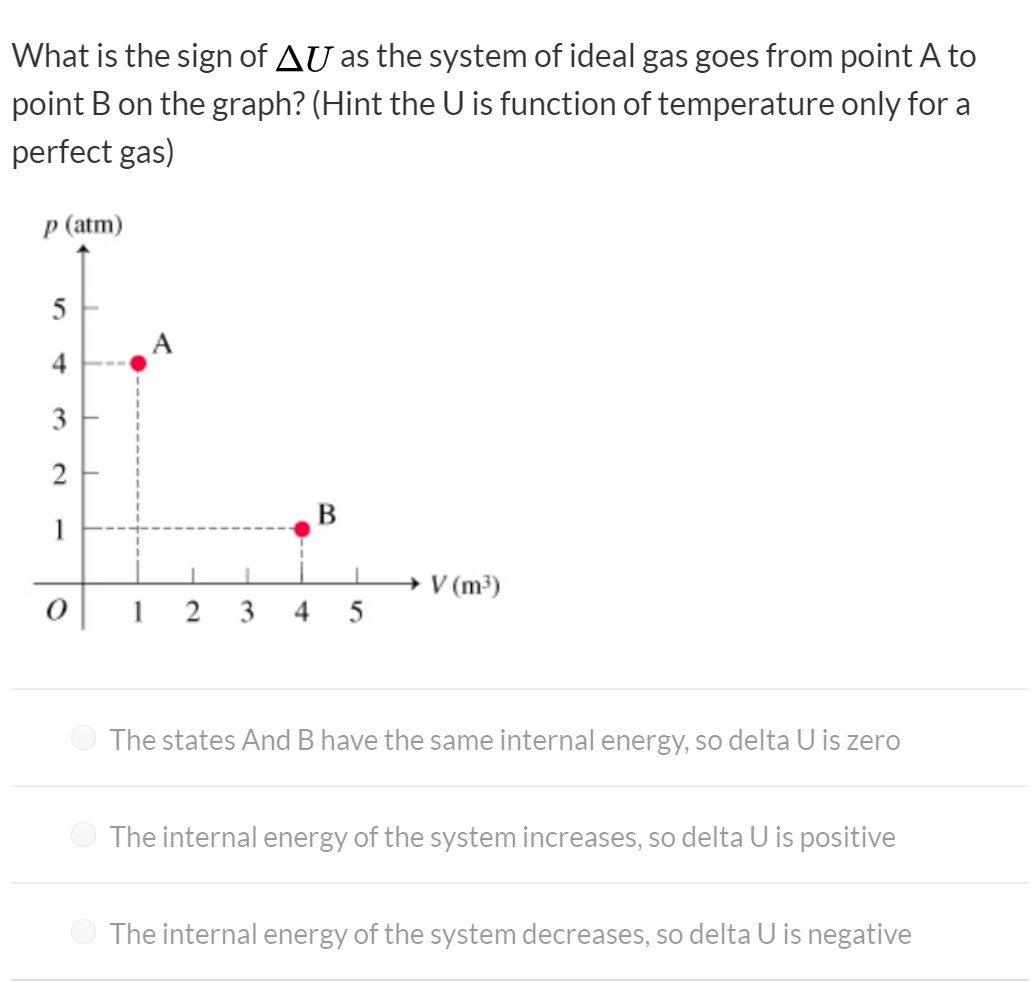What is the sign of AU as the system of ideal gas goes from point A to point B on the graph? (Hint the U is function of temperature only for a perfect gas) p (atm) 4 3 2 B V (m3) 1 2 3 4 The states And B have the same internal energy, so delta U is zero The internal energy of the system increases, so delta U is positive The internal energy of the system decreases, so...

• ### Learning Goal Internal Energy of an ideal gas The internal energy of a system is the...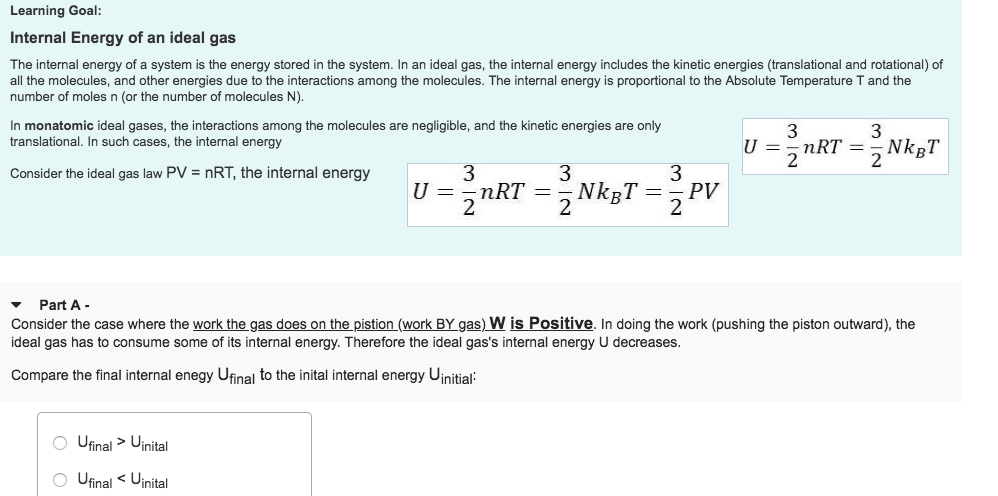Learning Goal Internal Energy of an ideal gas The internal energy of a system is the energy stored in the system. In an ideal gas, the internal energy includes the kinetic energies (translational and rotational) of all the molecules, and other energies due to the interactions among the molecules. The internal energy is proportional to the Absolute Temperature T and the number of moles n (or the number of molecules N). n monatomic ideal gases, the interactions among the molecules...

• ### Consider each of the following processes and determine if work is done by the system, work...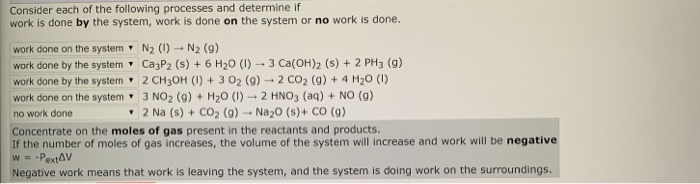Consider each of the following processes and determine if work is done by the system, work is done on the system or no work is done. work done on the system N2 (1) N2 (9) work done by the system Ca3P2 (s) + 6 H20 (1) + 3 Ca(OH)2 (s) + 2 PH3 (9) work done by the system' 2 CH3OH (1) + 3 02 (9) 2 CO2 (9) + 4H20 (1) work done on the system 3 NO2 (9)...

• ### Calculate the change in the internal energy of the system for a process in which the...

Calculate the change in the internal energy of the system for a process in which the system absorbs 140 J of heat from the surroundings and does 85 J of work on the surroundings.

• ### A gaseous system releases 23 J of heat and increases its internal energy by 60 J....

A gaseous system releases 23 J of heat and increases its internal energy by 60 J. Calculate the amount of work associated with this scenario. 83 J of work is done by the system on the surroundings. The system does no work on the surroundings. 37 J of work is done by the system on the surroundings. 83 J of work is done on the system by the surroundings. 37 J of work is done on the system by the...